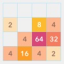Hlavní navigace

#MTP Merge 1.0

3

Pro hodnocení programu se prosím nejprve

Staženo
0 ×
Zdarma

## Sdílet

"MTP Merge" is a simple puzzle game. Swipe to move all tiles. When tiles with the "rule" number touch, they merge into one.

There are 3 modes:
2048 Classic:
* 2 +2 = 4
* 4 +4 = 8
* 8 +8 = 16
* 16 +16 = 32
* ....

3 Number:
* 3 + 3 + 3 = 9
* 9 + 9 + 9 = 27
* 27 + 27 + 27 = 81
* 81 + 81 + 81 = 243
* ....

Fibonacci:
* 2 + 3 = 5
* 3 + 5 = 8
* 5 + 8 = 13
* 8 + 13 = 21
* 13 + 21 = 34
* ....

There are 3 board:
* 3x3
* 4x4 (default)
* 5x5

"Merge Game: Fibonacci sequence" is a simple puzzle game. This game is built from the Fibonacci sequence. Swipe to move all tiles. When two tiles with the number touch, they merge into one.

* 2 + 3 = 5
* 3 + 5 = 8
* 5 + 8 = 13
* 8 + 13 = 21
* 13 + 21 = 34
* ....

There are 3 modes:
* 3x3
* 4x4
* 5x5

There are 3 Themes
* Default
* Vibrant
* Joyful

1.0

22. 10. 2019

0 ×

866 kB

Ano

• #### Podporované jazyky

• Angličtina
Zobrazit více
3

Pro hodnocení programu se prosím nejprve

Staženo
0 ×

## Podobné programy

TIP: Stahují se vám programy pomalu? Změřte si rychlost svého internetového připojení.